## Capturas de ecrã

•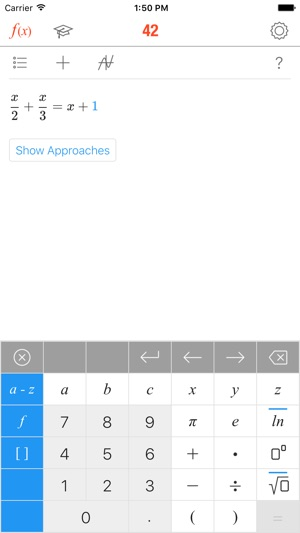•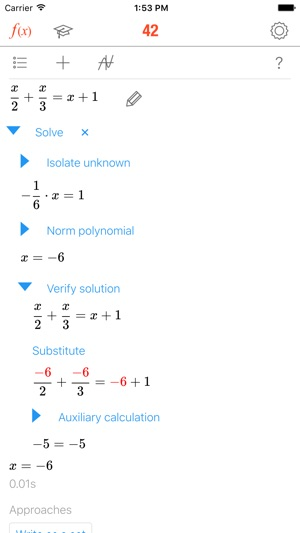•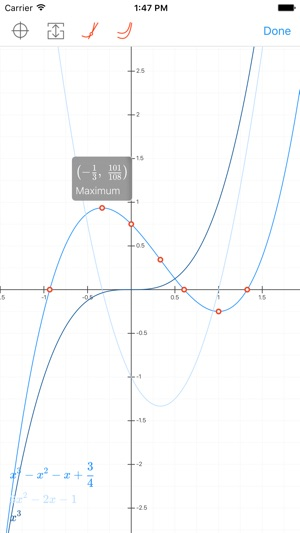•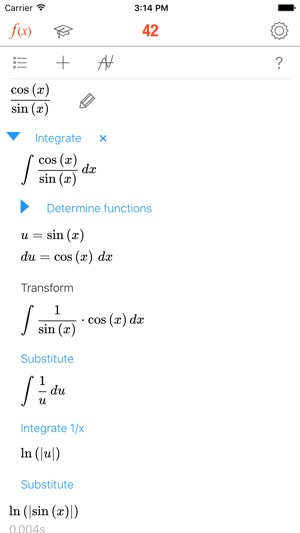•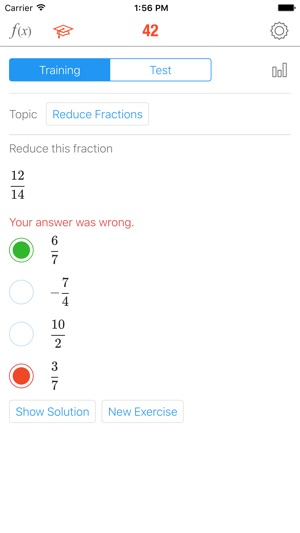## Descrição

With over 3.4 million downloads, MATH 42 is trusted worldwide by middle-school, high-school and college students to solve math problems, understand solutions, and get better grades. At a fraction of the cost of private tutors, MATH 42 delivers step-by-step solutions, intelligent approaches, and an Assessment Center for improving your skills.

TECHNOLOGY
• MATH 42 works without templates or pre-calculated solutions
• MATH 42 solves all problems on the fly, like a teacher at the board
• MATH 42 is a real symbolic algebra system
• MATH 42 is based on artificial intelligence

FEATURES
• Intuitive formula entry
• Calculator that instantly computes during input
• Interactive step-by-step solutions
• Suggestions on how to approach a problem
• Explanations of relevant math concepts with examples
• Interactive graphs to help visualize problems
• Assessment Center that fuels progress with tests and exercises

ASSESSMENT CENTER
• Tests and exercises created with educators and private tutors
• Training mode in which each problem can be analyzed step-by-step
• Test mode to identify strengths and weaknesses
• Results and statistics of progress over time

CAPABILITIES
Term transformation
• Simplifying and expanding
• Factorization (factoring out, applying binomial formulas)
• Transforming powers and roots
• Adding, multiplying, reducing and expanding fractions
• Computing the gcd and the lcm
• Trigonometric transformations
• Logarithmic transformations

Functions
• Roots
• Y-axis intersection
• Domain of definition
• Extremes (maximums, minimums)
• Inflexion points
• Curve sketching
• Derivation
• Fundamental derivatives
• Factor rule
• Sum rule
• Product rule
• Quotient rule
• Chain rule
• Integration
• Fundamental integrals
• Integration by substitution
• Integration by parts
• Partial fraction decomposition

Equations
• Linear equations
• Special equations of higher degree
• Linear systems of equations
• Non-linear system of equations

Matrices
• Multiplying matrices
• Transposing matrices
• Inverting matrices
• Determinants
• Characteristic polynomials
• Eigenvalues
• Eigenspaces
• Kernels
• Ranks
• Cross-product
• Gaussian elimination

Versão 3.3.24

MATH 42 — your digital private math tutor — is completely free now!
MATH 42 now supports iPhone X.

## Classificações e críticas

4.4 de 5
7 classificações

7 classificações

Mínimo retina ,

### Espetacular

Fantástica, ainda para mais grátis! É mesmo muito boa. Aconselho vivamente

mathpt ,

### Obrigatório registar

És obrigado a criares uma conta para usar o programa.

Marcolex ,

### 10 estrelas

Aplicação gratuita e muito funcional

## Informação

Fornecedor
Chegg, Inc.
Tamanho
70.4 MB
Categoria
Ensino

Requer o iOS 9.1 ou posterior. Compatível com iPhone, iPad e iPod touch.

Idiomas

Inglês

Classificação de 4+
Preço
Grátis

## Suporta

•